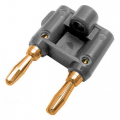# Is it possible to divide the current in a dc circuit and measure only half of the current?

#### injtsvetkov

Joined Dec 12, 2015
7
Hi everyone!
I need to measure direct current which is a bit higher than 10 amps, but my multimeter can measure maximum 10 amps. Is there a way to make current divider circuit (to split the current in 2) and measure the current on one branch, then derive the total current by multiplying the reading by 2?

#### crutschow

Joined Mar 14, 2008
33,358
You can put a resistance in parallel with, and equal to, the meter shunt resistance.

If you can't accurately measure that shunt resistance you could do the following:
Adjust the current limit on a power supply to its maximum (10A or less) and measure with your meter.
Then connect a resistance across it until the meter reads 1/2 that value.

I did a similar thing with a multimeter I had.
Since the meter shunt resistance is very low (perhaps 10mΩ if you meter has a minimum 100mV full scale setting), I used a steel paper clip for the added resistance.
I connected the paper clip wire between the two screw terminal connections in a dual banana plug (the two small openings below) and changed the wire length to adjust the resistance.
The banana jack is connected in parallel with the 10A current-measurement sockets on your multimeter.•DickCappels and injtsvetkov

#### injtsvetkov

Joined Dec 12, 2015
7

#### k1ng 1337

Joined Sep 11, 2020
826
You can put a resistance in parallel with, and equal to, the meter shunt resistance.

If you can't accurately measure that shunt resistance you could do the following:
Adjust the current limit on a power supply to its maximum (10A or less) and measure with your meter.
Then connect a resistance across it until the meter reads 1/2 that value.

I did a similar thing with a multimeter I had.
Since the meter shunt resistance is very low (perhaps 10mΩ if you meter has a minimum 100mV full scale setting), I used a steel paper clip for the added
I connected the paper clip wire between the two screw terminal connections in a dual banana plug (the two small openings below) and changed the wire length to adjust the resistance.
The banana jack is connected in parallel with the 10A current-measurement sockets on your multimeter.
I basically used your method to find the resistance of two 0.1ohm 10w resistors I bought since I don't have a precision ohm meter. Obviously the accuracy is only as good as my multimeter but is sufficient although I found an error between the shunt voltage and current through the meter. Is this error a direct variation (ohmic) between the two or does change at different voltage/currents?

I can rephrase my question if the above is confusing.

#### Ian0

Joined Aug 7, 2020
8,947
Easier to get a known shunt resistance, and simply use the mV range to measure the voltage across it.

#### crutschow

Joined Mar 14, 2008
33,358
I found an error between the shunt voltage and current through the meter. Is this error a direct variation (ohmic) between the two or does change at different voltage/currents?
The error is likely due to the various tolerances of the measurements.
How exactly did you do the measurement?

The only reason it would vary at different voltage/currents is if the resistance heated from the current and changed value due to the temperature change.